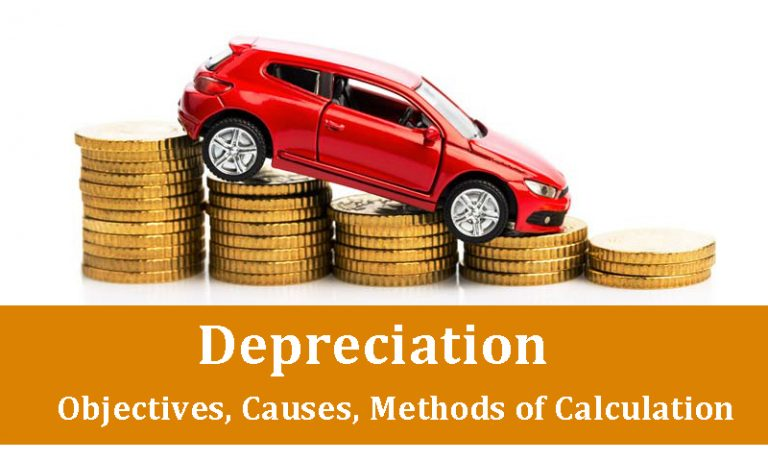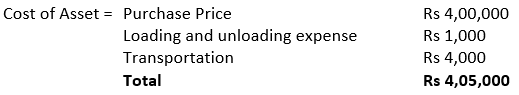# Depreciation: Objectives, Causes, Methods of CalculationDepreciation is the decrease in value of fixed assets due to wear and tear or technological obsolescence. All fixed assets depreciate except land which generally appreciates over the period of time.

The benefits of fixed assets are enjoyed by business over the years. The fixed assets provide benefits to the business for more than one year. These assets lose their usefulness due to wear and tear, change in technology etc. Therefore, these assets need to be replaced when their useful life is over. Therefore, expense incurred on purchase of fixed assets cannot be booked in one particular year. Instead, the expense is allocated over its useful life. Each year’s allocated expense is charged as depreciation expense for that year.

For example, an office table is purchased for Rs 5000 and it is estimated that after ten years it will be scrapped. The useful life of the table is ten years over which the cost of Rs 5000 will be distributed. By uniform distribution, Rs 500 is the depreciation expense for each year

The fixed assets are shown in balance sheet at their net values.

## Cause of Depreciation

The depreciation can be caused due to following reasons:

1. Wear and Tear: As we put the assets to use, its value decreases due to wear and tear. As the time passes, the natural elements like sunlight, rain also causes physical deterioration of assets.
2. Obsolescence: Due to improvement in technology, sometimes assets needs to be replaced even before they are actually worn out. The superior equipment allows good and services to be produced at lower cost. Thus, usage of old equipment becomes inefficient and costlier. Secondly, due to change in fashion, style, taste or market conditions; there is decline in demand for certain good and services. The assets engaged in production of such good and services need to be discarded. Thus, the loss of value of fixed assets due to such obsolescence is also charged as depreciation.## Objectives of Depreciation

The objectives of depreciation are:

• Retain funds for replacement of assets: If depreciation is provided, there is reduction in net profit due to booking of expense in profit and loss account. The business will be able to retain cash for replacement of assets with new assets. Thus, it helps in running the business smoothly and continuously.
• True financial position of business: If depreciation is not provided, the balance sheet will not present the true financial position of fixed assets. As fixed assets are worn out on year on year basis, the true value of fixed assets will be reflected in balance sheet. The profit and loss account will also present the true figure of net profit after deducting the depreciation expense.

## Factors Affecting the Depreciation

The factors affecting the amount of depreciation are:

1. Cost of Asset: The cost of asset includes purchase price of asset and expenses incurred before its first use.
2. Useful Life of Asset: The expected number of year for which asset will remain in use is called Useful life of Asset
3. Scrap Value: The scrap value is the residual value at which asset could be sold to scrap dealer after its useful life.
4. Depreciable value of Asset: Depreciable value of Asset is cost of asset minus Scrap value.

For example, a printing machine is purchased for Rs 4,00,000. Rs 1,000 was paid its loading and unloading. Rs 4,000 was paid for transportation to business premises. The printing machine is expected to run for 10 years and thereafter would be sold for Rs 40,000. Let’s calculate the depreciable value of asset.Thus cost of asset comes out to Rs 4,05,000

Useful life of printing machine = 10 years

Scrap value of printing machine = Rs 40,000

Depreciable value of printing machine = Rs 4,05,000 – Rs 40,000 = Rs 4,65,000

## Methods for calculation of Depreciation

There are various methods to calculate the depreciation. But two most important and commonly used methods are:

• Straight Line Method
• Reducing Balance Method

### Straight Line Method

This method is also called Fixed Installment Method or Original Cost Method. This is simple and very popular method of calculating depreciation. In straight line method of calculating the depreciation, the depreciation is charged on uniform basis from year to year. The fixed amount of depreciation is written off every year. It is calculated as:

Depreciation of each year = (Cost of Assets – Scrap Value of asset)/Number of years of expected life

For example, a machine is purchased for Rs 2,20,000 and that its useful life is 10 years. After its useful life its scrap value is Rs 20,000. Depreciation of one year can be calculated as:

Depreciation of one year = (2,20,000 – 20,000)/10 = 20,000

### Reducing Balance Method

This method is also called Diminishing Balance Method or Written Down value method. In this method of calculating the depreciation, the depreciation is charged at fixed percentage of asset value at beginning of each year. Under this method, the value of an asset never reaches zero value.

For example, the cost of the asset is Rs 50,000 and the percentage to be written off each year is 10%. In the first year the amount of the depreciation will be Rs 5,000 i.e., 10% of Rs 50,000. This will reduce the book value to Rs 45,000 i.e. Rs 50,000 – Rs 5,000. Now, at the beginning of the next year the book value is Rs 45,000. The amount of the depreciation for the next year will be Rs 4,500, i.e., 10% of Rs 45,000. Thus, every year the amount of the depreciation will go on reducing.